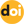The peer-reviewed scientific and technology journal. ISSN: 0204-3327

# Stochastic theory of zero power nuclear reactors part 3. Stochastic differential equations of zero-dimensional reactor kinetics. Weak external neutron source. Analysis of the equivalent reactivity noise modelhttps://doi.org/10.26583/npe.2014.3.11

### UDC: 621.039.516

Stochastic differential equations of zero-dimensional reactor kinetics have been derived assuming that the discrete-time and continuous-time branching processes should have moments of distribution which converge up to the second order, inclusive. The regular part of the equations derived is similar to that of the zero-dimensional reactor kinetics equations. The regular part is influenced by the totality of the Gaussian white noise inputs with appropriate coefficients.

An approximated distribution of the continuous-time branching process values has been derived by using a prompt neutron approximation from the one-dimensional equation for the characteristic function. Although this distribution may not have much physical significance, it allowed the concept of the weak external neutron source to be clarified more precisely.

An analysis has been made of the well-known Cohn’s stochastic model, with reactivity noise introduced by analogy with the Schottky effect. It has been shown that the model works well due to a very small fraction of delayed neutrons emitted by all the fissionable isotopes.

1. Volkov Yu.V. Stohasticheskaya teoriya yadernyh reaktorov nulevoj moschnocti. Chast’
2. Fizicheskaya i matematicheskaya modeli. [Stohchastic Theory of Zero Power Nuclear Reactors. Part 1. Physical and Mathematical Models]. Izvestiya vuzov. Yadernaya energetika. 2013, no. 4, pp. 127-134.
3. Tikhonov V.I., Mironov M.A. Markovskie processy [Markov Processes]. Moscow, Sovetskoe Radio Publ., 1977 (in Russian).
4. Korolyuk V.S., Portenko P.I., Skorokhod A.V., Turbin A.F. Spravochnik po teorii veroyatnostej i matematicheskoj statistike [Probability Theory and Mathematical Statistics. Handbook]. Moscow, Nauka Publ., 1986 (in Russian).
5. Sudek G. Problemy kinetiki reaktora. V sb. Teoriya yadernyh reaktorov [Aspekts of Reactor Kinetics. In Reactor Theory]. Moscow, Gosatomizdat Publ., 1963 (in Russian).
6. Hansen G.E. Assembly of Fissionable Material in the Presence of a Weak NeutronSource. Nucl.Sci. Eng. 1960, v. 8, pp. 709-719.
7. Koks D.R., Smit V.L. Teoriya vosstanovleniya [Recovering Theory]. Moscow, Sovetskoe Radio Publ., 1967 (in Russian).
8. Hankins D.E. Effect of Reactivity Addition Rate and of Weak Neutron Source on the Fission Yield of Uranium Solutions. Nucl. Sci. Eng. 1966, v. 26, pp. 110-116.
9. Urig R. Statisticheskie metody v fizike yadernyh reaktorov [Statistical Methods in Reactor Physics]. Moscow, Atomizdat Publ., 1974 (in Russian).